# 15. Exercises.

3.1) A functionalwhere V  is a normed linear space, is linear if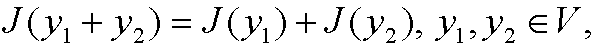andWhich of the following functionals on C1[a,b] are linear?

a)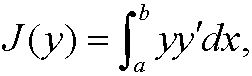b)c)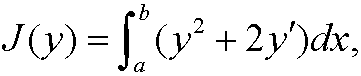d)3.2) Show that the Euler equation for the functional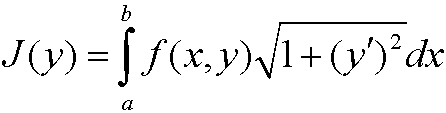has the form3.3) Find an extremal to3.4) Find the extremals to3.5) Find the extremals to

a)b)3.6) Show that the Euler equation for the surface area functionalis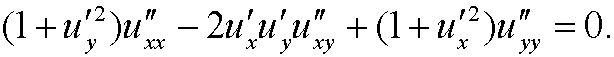3.7) Find an extremal towww.larserikpersson.se